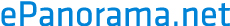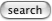# Noise types

## White noise

White noise has equal power density across the entire spectrum (per Hz basis). White Noise has constant energy at all frequencies.

"white noise" was named such because it has an equal amount of power per Hz (or whatever your unit frequency) just like white light. true white noise (with infinite bandwidth) cannot exist since it has infinite power.

White noise is noise in which the intensity of the power spectral density is constant and independent of frequency. It is equivalent to saying that the signal power for a constant bandwidth centred at frequency fo, does not change if fo is varied.

## Pink noise

Pink noise has uniform power density for a relative bandwidth (octave, decade, you-name-it). Pink noise has constant energy per constant percentage bandwidth. This equates to a -3dB/octave frequency response.

It's easy to turn white noise into pink noise by running it through a 3 dB/octave filter.

For example, an octave filter centered at 1kHz has half the bandwidth of an octave filter centered at 2kHz. If pink noise is driven into each filter with the same level, the output of each filter would be the same.

At low frequencies, it got the name 1/f noise, especially when we were desperately trying to understand and eliminate it from transistors. We eventually found that it was inherent in the physics of silicon, and that, while we could lower its frequency of onset, it is here to stay. The frequency was eventually pushed so low that it came to be called "popcorn noise". Hook a pair of 3000 ohm heasphones to the output of an opamp running wide open, and you'll hear that the name fits.

Pink noise is the common name given to 1/f noise which crops up in many natural stochastic processes and approximates the power spectrum of music signals too. As the name suggests the intensity of the pink noise power spectral density is proportional to 1/f, the frequency. An interesting characteristic of such a noise signal is that the noise power for exponentially increasing bandwidths is constant. What this means is that given a bandpass filter with a constant Q (ratio of centre frequency and bandwidth) the output power of the filter fed by 1/f noise is constant irrespective of the centre frequency. Hence the use of 1/f (pink noise) sources in combination with audio graphic equalisers and octave band or third octave band real time analysers.

Isn't it also true that white noise is entirely uncorrelated whereas pink noise is correlated over short time scales? the fact that the power spectrum of colored noise is not flat across the entire frequency spectrum automatically implies this. you should recall: autocorrelation in time <-- Fourier --> PSD in frequency therefore if the noise is not white you will not get a delta function in time autocorrelation, therefore there will be some correlation.

### Where did pink noise name came from ?

"red noise" got its name after a connection with red light which is on the low end of the visable light spectrum. red noise is white noise run through an integrator (with 1/(jf) frequency response or -6 dB/octave) so low frequencies are emphasized and highs are attenuated. the power spectrum of red noise is proportional to the square of the magnitude of the filter ( 1/(f^2) ).

"pink noise" (a.k.a. "flicker noise" or "1/f noise") is halfway between white noise and red noise. so its rolloff is at a -3 dB/octave rate. pink noise filters are a little harder to design but i had posted a design that requires 3 poles and 3 zeros that seems to do okay. to find it, go to DejaNews and search comp.dsp for the term "pink noise" or "1/f noise".